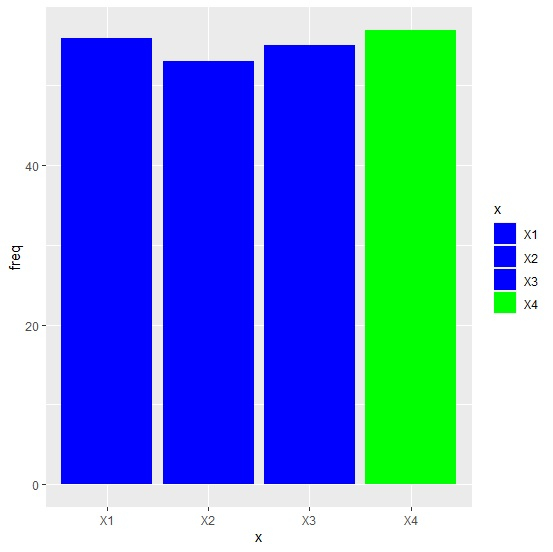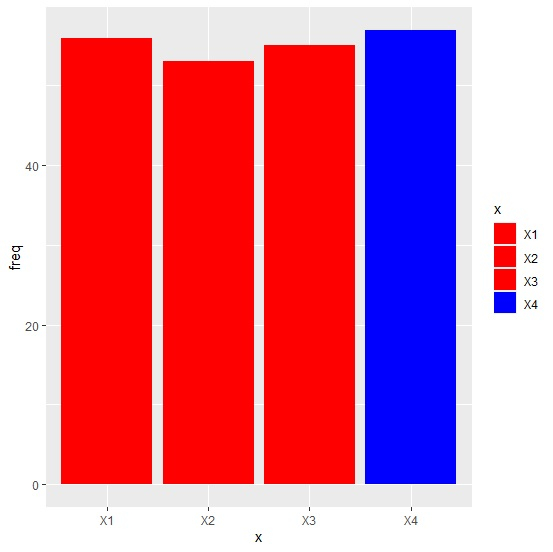# How to create a barplot with one of the bars having different color in R?

A bar plot represents discrete data and the bars in the bar plot are usually of same color but we might want to highlight a particular bar based on the characteristics of the data or the objective of the analysis project. For example, if a particular bar represents highly severe situation or highly unimportant situation then we can change the color that particular bar so that people can easily point out that bar.

Consider the below data frame −

## Example

Live Demo

x<-c("X1","X2","X3","X4")
freq<-c(56,53,55,57)
df<-data.frame(x,freq)
df

## Output

x freq
1 X1 56
2 X2 53
3 X3 55
4 X4 57

Loading ggplot2 package and creating bar plot with green color for bar showing 57 −

## Example

library(ggplot2) ggplot(df,aes(x,freq,fill=x))+geom_bar(stat="identity")+ scale_fill_manual(values=c("blue","blue","blue","green"))

## OutputCreating bar plot with blue color for bar showing 57 −

## Example

ggplot(df,aes(x,freq,fill=x))+geom_bar(stat="identity")+ scale_fill_manual(values=c("red","red","red","blue"))

## Output